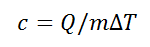# Specific Heat calculator

This CalcTown calculator calculates the specific heat of a substance.

K
J
Kg

#### Result

J/Kg-Kwhere

c: Specific Heat , in J/(kg.K)
Q: Heat required for the temperature change, in J
ΔT: Temperature change, in K
m: Mass of the object, in kg